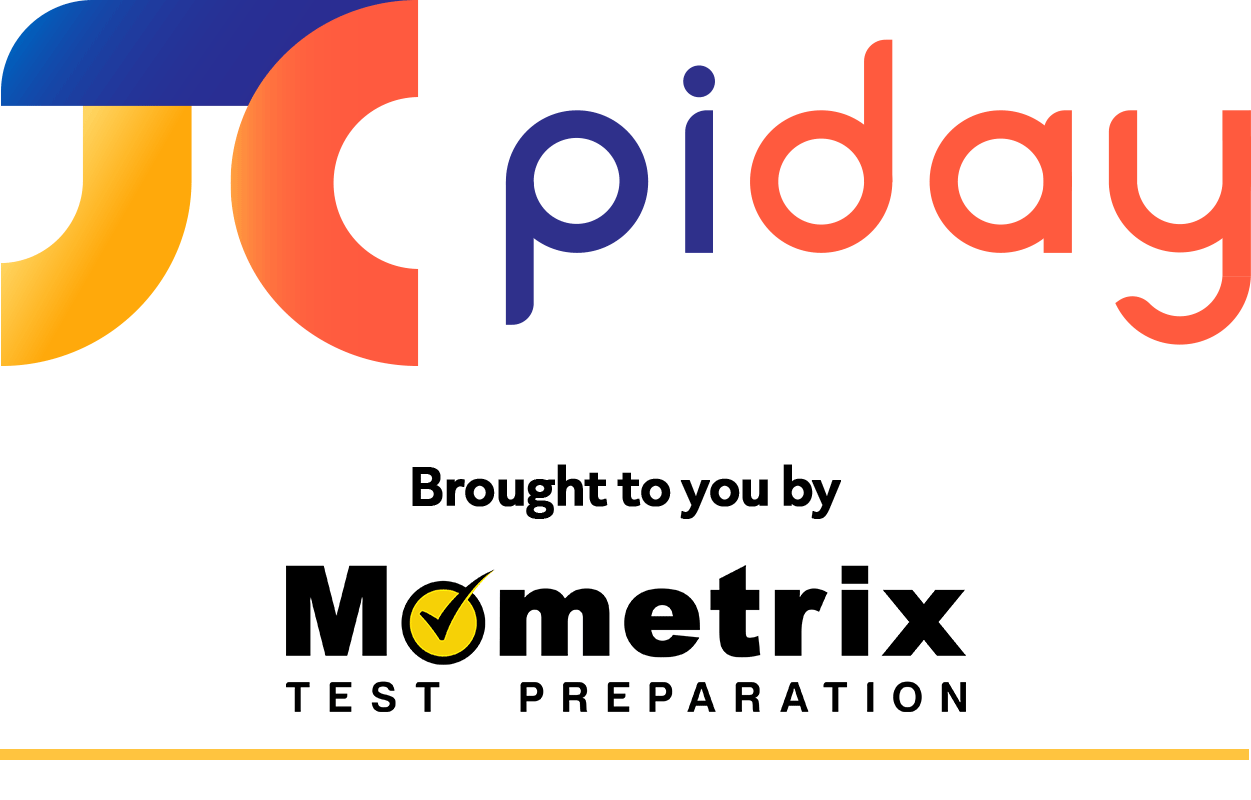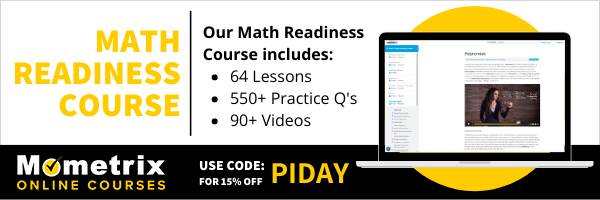## What is math?

Math is a shortened form of the word mathematics. Mathematics is the study of numbers, quantities, structure, and space.

## What is mean?

Mean is another word for average. Finding the mean is finding the central point in a set of numbers. To do this, add up all your values and then divide by the number of values you are given.

Ex. Find the mean of the set: {100, 98, 89, 92, 81}.

$$\large \frac{100+98+89+92+81}{5}=\frac{460}{5}=92$$

The mean of this set is 92.

## What is mode?

Mode is the most frequently occurring value in a set of data. It is possible to have more than one mode in a set.

Ex. What is the mode of the set: {11, 13, 21, 11, 19, 13, 11, 2}?

The mode is 11 because it is included three times in the set, while every other number is included only once or twice.## What is range?

Range is the distance between the largest and smallest values in a set of numbers.

Ex. Find the range of the set: {3, 11, 19, 1, 7}.

Largest Number: 19

Smallest Number: 1

19 – 1 = 18

The range of this set of numbers is 18.

## What is pi?

Pi (π) is a Greek letter that represents the ratio of a circle’s circumference to its diameter. It is a never-ending number that cannot be written as a fraction, which means it is irrational. The approximate value of pi is 3.14.

## What is a function?

A function is a relation between inputs and outputs in which every input has exactly one output.

You can tell if an equation is a function by graphing it and performing the vertical line test. To do this, imagine a vertical line is traveling across the x-axis. If at any x-value the line hits two different points, or y-values, your equation has failed the vertical line test and is not a function.

Ex. y = x is a function because for every x-value there is exactly one y-value.

## What does product mean?

The product of numbers is the answer you get when you multiply those numbers together. In other words, a product is an answer to a multiplication problem.

Ex. What is the product of 3 and 4?

3 x 4 = 12

The product of 3 and 4 is 12.

## What is a variable?

A variable is a symbol, typically an English or Greek letter, that represents an unknown value.

Ex. In the expression: $$x^{2}+2y-3z$$, x, y, and z are all variables.

## What is a constant?

A constant is an unchanging number, or a value that does not change.

Ex. In the expression: $$3x^{2}+4y+5z$$, 3, 4, and 5 are all constants.

## What is circumference?

Circumference is the perimeter of a circle. It can be found by multiplying the circle’s diameter by pi, or by doubling its radius and then multiplying by pi.

C = πd                     or                     C = 2πr

Ex. What is the circumference of a circle with a radius of 5 cm?

C = 2πr

C = 2π(5 cm)

C = 10π cm ≈ 31.4 cm